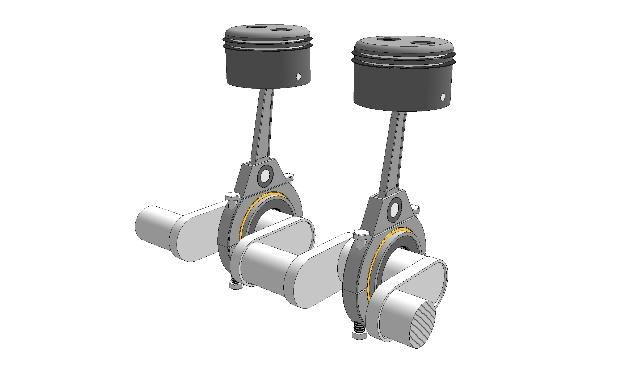# Activity 4 Modeling Automobile Pistons

 Date 20.05.2018 Size 84.26 Kb.

Name: Date: Page of

Activity 6.5.4 Modeling Automobile Pistons

Although many components go into making a car’s engine produce power, one of the most important parts are the pistons. Pistons allow an engine to pull in cool, dense air that is mixed with the car’s fuel. That fuel and air mixture is then compressed by the piston and ignited by the sparkplug to produce the energy needed to power the car. Pistons are mounted on a crank shaft that spins in a circular motion, pushing the pistons up and down hundreds or even thousands of times each second. One video you can watch to see how pistons work is the You Tube video “Four Stroke Engine: How it Works” by blenderguy2008 at https://www.youtube.com/watch?v=OGj8OneMjekhttps://sketchygraphics.wordpress.com/sgb-gallery/2piston/

The height of the top, or heads, of the pistons can be approximately modeled by a sinusoidal equation. You can read parts of the Wikipedia article “Piston Motion Equations” to learn why the motion is asymmetric and not exactly a sine function https://en.wikipedia.org/wiki/Piston_motion_equations . For the purpose of this exercise we will assume that the model for height of the piston head in its cylinder is a sinusoidal function of time. Sara is a teenager who is intrigued by both engines and mathematics. In her automotive class she was doing some research on piston timings and came across a book that showed the location of the piston heads for particular engines at any given time. She finds her car’s engine in the book and begins to analyze the given table.

The time units are stated to be in tenths of a second, so .1 is representative of one-tenth of one-tenth of a second, or one-hundredth of a second. Height is given in inches. Negative heights represent that the head of the piston is located below its average height.
 Time(t) 0.1 0.5 0.7 1 1.2 1.5 1.9 2.2 Height (h) 5.45 9.14 8.32 3.68 -0.96 -1.78 1.91 7.05

a) On the graph paper provided, plot the given points (time, height) for the piston. Make sure to label the axes and choose an appropriate scale for the given data. Then, draw a curve that models the periodic motion of the piston.

b) Assume that the height of a piston as a function of time can be approximated by a sinusoidal function. Determine a trigonometric equation that models the relationship shown in your graph for part a.

c) When a mechanic refers to a piston “firing”, they are referring to the process that starts with air being drawn in, followed by the compression and ignition of the air/fuel mixture and ending with the return of the piston to its initial position. How long will it take for Sara’s engine to fire 25 times? Show how you got your answer, rounding to the nearest tenth.

d) If her engine is operating properly, what should the piston height be at the following times?

Show work, rounding your answer to the nearest hundredth of an inch. Remember that x is measured in tenths of a second.

1. 0.8 of a second?

1. 0.08 of a second?

(+) Bonus Question: In a four-cylinder engine, four pistons operate simultaneously to produce energy. To make sure that the car will run smoothly the pistons fire at different times. Provide three additional equations that could be used to model the movement of the additional three pistons. Then, give a brief description as to why your equations would work in this scenario.

Activity 6.5.4 Connecticut Core Algebra 2 Curriculum Version 3.0